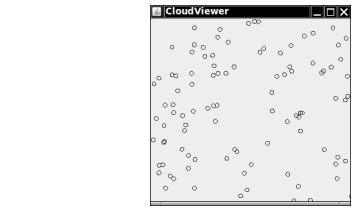Implement a class Cloud that contains an array list of Point2D.Double objects. Support methods

public void draw(Graphics2D g2)

Draw each point as a tiny circle.

Write a graphical application that draws a cloud of 100 random points.

Here is a sample program output:Complete the following file:

### Cloud.java

Use the following files:

CloudComponent.java

```import javax.swing.JComponent;
import java.awt.Graphics;
import java.awt.Graphics2D;
import java.awt.geom.Point2D;
import java.util.Random;

public class CloudComponent extends JComponent
{
public void paintComponent(Graphics g)
{
Graphics2D g2 = (Graphics2D) g;

Cloud c = new Cloud();
Random generator = new Random();
double x = 0;
double y = 0;

for (int i = 0; i < 100; i++)
{
x = getWidth() * generator.nextDouble();
y = getHeight() * generator.nextDouble();
}

c.draw(g2);
}
}
```

CloudViewer.java

```import javax.swing.*;

/**
Displays a cloud of circles.
*/
public class CloudViewer
{
public static void main(String[] args)
{
JFrame frame = new JFrame();

final int FRAME_WIDTH = 300;
final int FRAME_HEIGHT = 300;

frame.setSize(FRAME_WIDTH, FRAME_HEIGHT);
frame.setTitle("CloudViewer");
frame.setDefaultCloseOperation(JFrame.EXIT_ON_CLOSE);

CloudComponent component = new CloudComponent();